## Outliers — Friends or Foes?

If you are an experimental working person, I guess you have already faced situations with outliers, i.e. any data points appearing out of range compared to the others.

Probably you might have expected a clearer, maybe even more mathematical, definition of the term outlier. In fact, there are various definitions of this term in the literature, some are more mathematical, others are rather as general as mine. However, I think it is impractical to give one definition covering all possible situations. One question is how to define when a data point is out of range. The other question is, given that there is a way to detect outliers, what to do with them? Simply remove them without any thoughts? This is clearly not the best practice since outliers might contain useful information. They might for instance contain information about wrong instrument settings or unrecognized noise sources. Thus, they can be regarded as your friends. Expecting data to be distributed in a certain way (e.g. normal) can also lead to falsely detected outliers. E.g. if data is lognormally and not normally distributed, data points can emerge that seem to be outliers when wearing the normal distribution glasses but not when wearing the lognormal distribution glasses. If there are reasonable assumptions about the underlying distribution of the data, there exist a bunch of different statistical tests to identify outliers (for a good overview refer to [Iglewicz1993]). Most commonly data is assumed to be normally distributed. There exist outlier tests like the Grubbs-test in order to identify them. The test is basically a hypothesis test whose null hypothesis is that  follows the same distribution as the other data points and thus, belong to the same population. If the null hypothesis is rejected the suspicious data point is an outlier. The Grubbs-test requires the mean  and standard  deviation of the data set and then calculates the z-score:Hereindenotes the suspicious data point (i.e. the minimum or maximum of the ordered data set). If the z-score is greater than a threshold value the data point can be regarded as an outlier (i.e. the null hypothesis is rejected). As is typical for a hypothesis test, the user-defined threshold depends on the confidence level, i.e. the probability that the data point is falsely rejected. The threshold can either be deduced from an appropriate Grubbs table or can be calculated according to: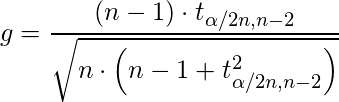Where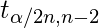denotes the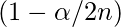-percent quantile of Student’s t-distribution with  degrees of freedom (n: # of data points). In Excelcan be calculated using T.INV(1-α/(2n), n-2). By the way, the minus two term for the degrees of freedom, , arises because one degree is used up by the mean and the other is used up by the standard deviation calculation.

The Grubbs-test is designed to identify a single outlier in a dataset. But other tests like the generalized extremized student deviate (GESD) test exist that can detect multiple outliers at once and shall be used to identify multiple outliers that otherwise mask each other. For the GESD, a suspected number r of outliers need to be given into the analysis and then some sort of sequential Grubbs-test is performed checking the r y-values that give the biggest Grubbs value g. Using the mean and the standard deviation as in the aforementioned tests can be unfortunate especially if multiple outliers are present at one end. In this case  and  are badly “squeezed” by the outliers which might lead to the inability even to detect one outlier. Thus, it might help using robust alternatives to the mean and standard deviation, namely the median  and the median absolute deviation (MAD). Then using those in the z-score equation gives: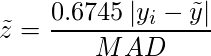If this modified z-score is greater than some threshold d, the value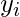is an outlier. Based on simulation studies Iglewicz and Hoaglin suggested to choose d = 3.5.

Another useful tool in order to identify outliers is a box-plots. A typical box-plot summarizes the quartiles of a data in terms of a box with the lower quartile (i.e. 25 %, Q1) at the bottom, the upper quartile (75 %, Q3) forming the top of the box and the median inside of the box (typically indicated by a line). Whiskers emerge below and above the box whose ends indicate certain values of the data set. Tukey for instance used plus and minus 1.5 time the interquartile range (IQR = Q3-Q1) for the upper and lower whisker, respectively. This, to my knowledge, is the most common type of box-plot  (Tukey is actually the inventor of box-plots). All data points that extend above (or below) 1.5 x IQR might be outliers. These data points are plotted individually in the box-plot.

### Dr. Mario Schneider

Mario is an analytical chemist with a strong focus on chemometrics and scientific data analysis. He holds a PhD in biophysics and is the author of a book on data analysis for scientists. In his spare time he is a MATLAB, R, Excel and Python enthusiast and experiments with machine learning algorithms.